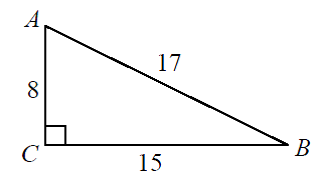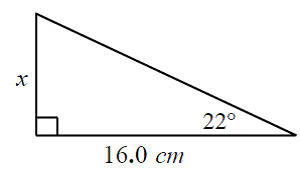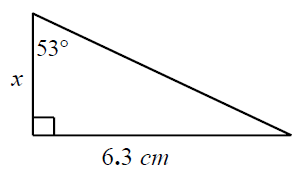# Use tangent ratio to calculate angles and sides (Tan = $\frac{o}{a}$ )

### Use tangent ratio to calculate angles and sides (Tan = $\frac{o}{a}$ )

Tangent ratio is yet another trigonometric ratio for right-angled triangles. Tangent ratio is the ratio of opposite side to adjacent side of a right triangle. Same as the sine and cosine ratios, tangent ratios can be used to calculate the angles and sides of right angle triangles.

#### Lessons

• Introduction
What are sine, cosine and tangent?

• 1.
Determine each tangent ratio using a calculator
i) $\tan 70^\circ$
ii) $\tan-134^\circ$

• 2.
Determine the angle to the nearest degree using a a calculator
i) $\tan \theta = 0.64$
ii) $\tan \theta = -1.45$

• 3.
Determine the angles and sides using Tangent
a)
Find angle A and B:b)
Find the value of "x" using tangentc)
Find the value of "x" using tangent• 4.
Draw and label a right triangle to illustrate tangent ratio, then calculate the angle.
a)
$\tan \theta = {5 \over 9}$